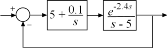# hasInternalDelay

Determine if model has internal delays

## Syntax

``B = hasInternalDelay(sys)``
``B = hasInternalDelay(sys,'elem')``

## Description

example

````B = hasInternalDelay(sys)` returns 1 (true) if the model `sys` has internal delays, and 0 (false) otherwise. If `sys` is a model array, then `B` is true if least one model in `sys` has delays.```
````B = hasInternalDelay(sys,'elem')` checks each model in the model array `sys` and returns a logical array of the same size as `sys`. The logical array indicates which models in `sys` have internal delays.```

## Examples

collapse all

Build a dynamic system model of the following closed-loop system.```s = tf('s'); G = exp(-2.4*s)/(s-5); C = pid(5,0.1); sys = feedback(G*C,1);```

Check the model for internal delays.

`B = hasInternalDelay(sys)`
```B = logical 1 ```

The model, `sys`, has an internal delay because of the transport delay in the plant `G`. Therefore, `hasInternalDelay` returns 1.

## Input Arguments

collapse all

Model or array to check for internal delays, specified as a dynamic system model or array of dynamic system models.

## Output Arguments

collapse all

Flag indicating presence of internal delays in input model or array, returned as a logical value or logical array.

## Version History

Introduced in R2013a